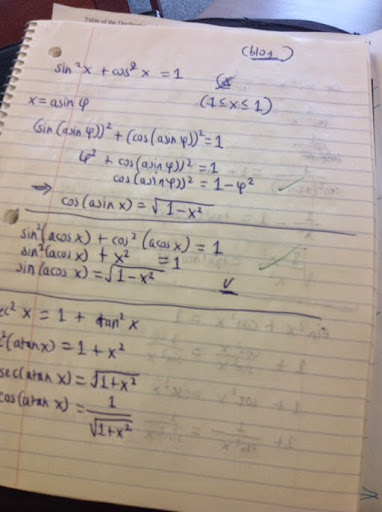## Friday, October 17, 2014

### sin(atan x), sin(acos x), cos(asin x), cos(atan x), tan(asin x), tan(acos x)

Deriving trig functions where the angle is an inverse trig function. Example: sin(acos x).

Inverse Functions

sin(asin t) = t
cos(acos t) = t
tan(atan t) = t

Other such calculations can be derived from the three Pythagorean trig identities:

sin^2 x + cos^2 x = 1
sec^2 = 1 + tan^2 x
1 + cot^2 x = csc^2 x

sin^2 x + cos^2 x = 1

Also known as (sin x)^2 + (cos x)^2 = 1

Let x = asin t where -1 ≤ t ≤ 1
(sin(asin t))^2 + (cos(asin t))^2 = 1
t^2 + (cos(asin t))^2 = 1
(cos(asin t))^2 = 1 - t^2
cos(asin t) = √(1 - t^2)

Let x = acos t, -1 ≤ t ≤ 1
(sin(acos t))^2 + (cos(acos t))^2 = 1
(sin(acos t))^2 = 1 - (cos(acos t))^2
(sin(acos t))^2 = 1 - t^2
sin(acos t) = √(1 - t^2)

sec^2 x = 1 + tan^2 x

sec^2 x = 1 + tan^2 x
1/cos^2 x = 1 + tan^2 x

Let x = atan t, -1 ≤ t ≤ 1

1/(cos(atan t))^2 = 1 + (tan(atan t))^2
1/((cos(atan t))^2 = 1 + t^2
(cos(atan t))^2 = 1/(1 + t^2)
cos(atan t) = √(1/(1 + t^2))

Let x = acos t, -1 ≤ t ≤ 1

1/(cos(acos t))^2 = 1 + (tan(acos t))^2
1/t^2 = 1 + (tan(acos t))^2
(tan(acos t))^2 = 1/t^2 - 1
(tan(acos t))^2 = (1 - t^2)/t^2
(tan(acos t)) = √(1 - t^2)/t

1 + cot^2 x = csc^2 x

1 + cot^2 x = csc^2 x
1 + 1/tan^2 x = 1/sin^2 x

Let x = asin t, -1 ≤ t ≤ 1

1 + 1/(tan(asin t))^2 = 1/(sin(asin t))^2
1/(tan(asin t))^2 = 1/t^2 - 1
1/(tan(asin t))^2 = (1 - t^2)/t^2
(tan(asin t))^2 = t^2/(1 - t^2)
tan(asin t) = t/√(1 - t^2)

Let x = atan t, -1 ≤ t ≤ 1

1 + 1/(tan(atan t))^2 = 1/(sin(atan t))^2
1 + 1/t^2 = 1/(sin(atan t))^2
(t^2 + 1)/t^2 = 1/(sin(atan t))^2
(sin(atan t))^2 = t^2/(t^2 + 1)
sin(atan t) = t/√(t^2 +1)

Summary:

cos(asin t) = √(1 - t^2)
sin(acos t) = √(1 - t^2)
cos(atan t) = √(1/(1 + t^2))
tan(acos t) = √(1 - t^2)/t
tan(asin t) = t/√(1 - t^2)
sin(atan t) = t/√(t^2 +1)

This blog is property of Edward Shore. 2014